Go back to Home

# Backgroud

I recently visited my brother in California and fell in love with it! The beautiful landscape and the energetic vibe really caught my attention. And obviously, you find some of the best companies in the world here in Silicon Valley! It just got me wondering- how much would it take to own a house here?

# Data

I started exploring a couple of housing datasets. Zillow keeps an impresive amount of data, but I found it to be a bit of overkill for the purpose of this analysis. I found this introductory dataset on Kaggle derived from the California census apt for my exploratory analysis

The data pertains to the houses found in a given California district and some summary stats about them based on the 1990 census data

The dataset has the following columns:

1. longitude: A measure of how far west a house is; a higher value is farther west
2. latitude: A measure of how far north a house is; a higher value is farther north
3. housingMedianAge: Median age of a house within a block; a lower number is a newer building
4. totalRooms: Total number of rooms within a block
5. totalBedrooms: Total number of bedrooms within a block
6. population: Total number of people residing within a block
7. households: Total number of households, a group of people residing within a home unit, for a block
8. medianIncome: Median income for households within a block of houses (measured in tens of thousands of US Dollars)
9. medianHouseValue: Median house value for households within a block (measured in US Dollars)
10. oceanProximity: Location of the house w.r.t ocean/sea

### Libraries

These were the libraries that were used during this analysis. I used "raster" library to crustruct the map of california and "ggplot2" to plot stacked graphs.

``````library(raster)
library(ggplot2)``````

### Data import

``````fpath<-"/Users/priyanshumadan/Desktop/housing.csv"
dim(all_housing)``````

### Data Cleaning

I did some basic data cleaning.I replaced N/A values with 0. Though NA were only in the "total_bedroom" column.

``all_housing\$total_bedrooms[is.na(all_housing\$total_bedrooms)]=0``

Alright time for some analysis!

# Basic Exploration

I initiated the analysis by exploring some basic summary metrics.

1. Total Households

``````dim(all_housing)
sum(all_housing\$households)``````

There are 10,310,499 households at 20,640 different locations across California.

2. Households by Ocean Proximity

``````# MAP CREATION

states    <- c('California')
us.states <- us[us\$NAME_1 %in% states,]
us.bbox <- bbox(us.states)
xlim <- c(min(us.bbox[1,1]),max(us.bbox[1,2]))
ylim <- c(min(us.bbox[2,1]),max(us.bbox[2,2]))

# CREATION OF DATA FRAME TO STORE HOUSEHOLD COUNT BY OCEAN PROXIMITY

household_ocean <- data.frame(matrix(nrow = 5, ncol = 2))
colnames(household_ocean) <- c("Ocean Proximity","Household Count")

household_ocean[,1]<-c("<1H OCEAN","INLAND","ISLAND","NEAR BAY","NEAR OCEAN")
household_ocean[,2]<-c(sum(all_housing\$households[all_housing\$ocean_proximity=="<1H OCEAN"])
,sum(all_housing\$households[all_housing\$ocean_proximity=="INLAND"])
,sum(all_housing\$households[all_housing\$ocean_proximity=="ISLAND"])
,sum(all_housing\$households[all_housing\$ocean_proximity=="NEAR BAY"])
,sum(all_housing\$households[all_housing\$ocean_proximity=="NEAR OCEAN"])
)

# PLOTTING OF BAR GRAPH

barplot( height = household_ocean[,2]
, las = 1
, col = cool_colour\$Coolness_Color
, border = NA  # eliminates borders around the bars
, main = "Households by Ocean Proximity"
, ylab = "count of households"
, xlab = "proximity"
, ylim = c(0,5000000)
,names.arg = household_ocean[,1]
)

# PLOTTING LOCATION POINTS ON THE MAP

plot(us.states, xlim=xlim, ylim=ylim)
points(all_housing\$longitude[all_housing\$ocean_proximity=="<1H OCEAN"], all_housing\$latitude[all_housing\$ocean_proximity=="<1H OCEAN"], col = "#F8756D", cex = .5)
points(all_housing\$longitude[all_housing\$ocean_proximity=="NEAR BAY"], all_housing\$latitude[all_housing\$ocean_proximity=="NEAR BAY"], col = "#00BE7D", cex = .5)
points(all_housing\$longitude[all_housing\$ocean_proximity=="INLAND"], all_housing\$latitude[all_housing\$ocean_proximity=="INLAND"], col = "#A3A500", cex = .5)
points(all_housing\$longitude[all_housing\$ocean_proximity=="ISLAND"], all_housing\$latitude[all_housing\$ocean_proximity=="ISLAND"], col = "#03B0F6", cex = .5)
points(all_housing\$longitude[all_housing\$ocean_proximity=="NEAR OCEAN"], all_housing\$latitude[all_housing\$ocean_proximity=="NEAR OCEAN"], col = "#E76AF3", cex = .5)``````
• First few lines of code is for the creation of the california map. Any number of states can be added just by including the name of the state in the state varable.
• I then constructed a dataframe (households_ocean) so as to store the count of households by ocean proximity. This makes the data easier to understand as I wont have to go back to the original dataset.
• Finally comes the plotting of location points on the map. First step was to plot the map of california using "plot" function followed by plotting the points using "points" function and using "latitude" and "longitude" column as attributes for those points.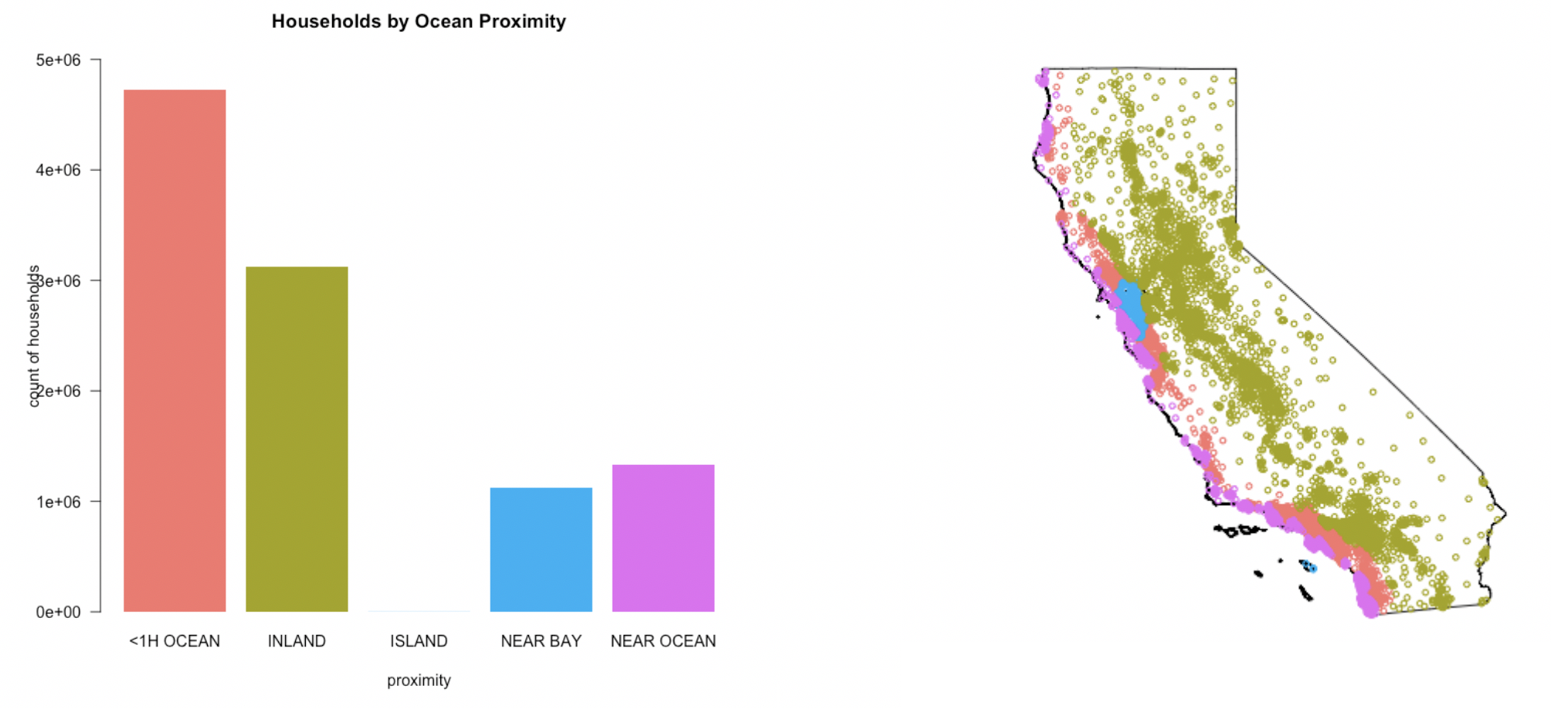It can be observed from the graph above (Households by Ocean Proximity) that there are more households (4,730,118 households) situated 1 hour away from ocean (<1H OCEAN) as compared to other areas. It is followed by households (3,127,759) that are situated inland (INLAND ). Though it can be seen from the map that INLAND households are much more widespread as compared to that of other locations making areas closer to the ocean much more densily populated as compared to INLAND.

Lets confirm this hypothesis by looking at the population data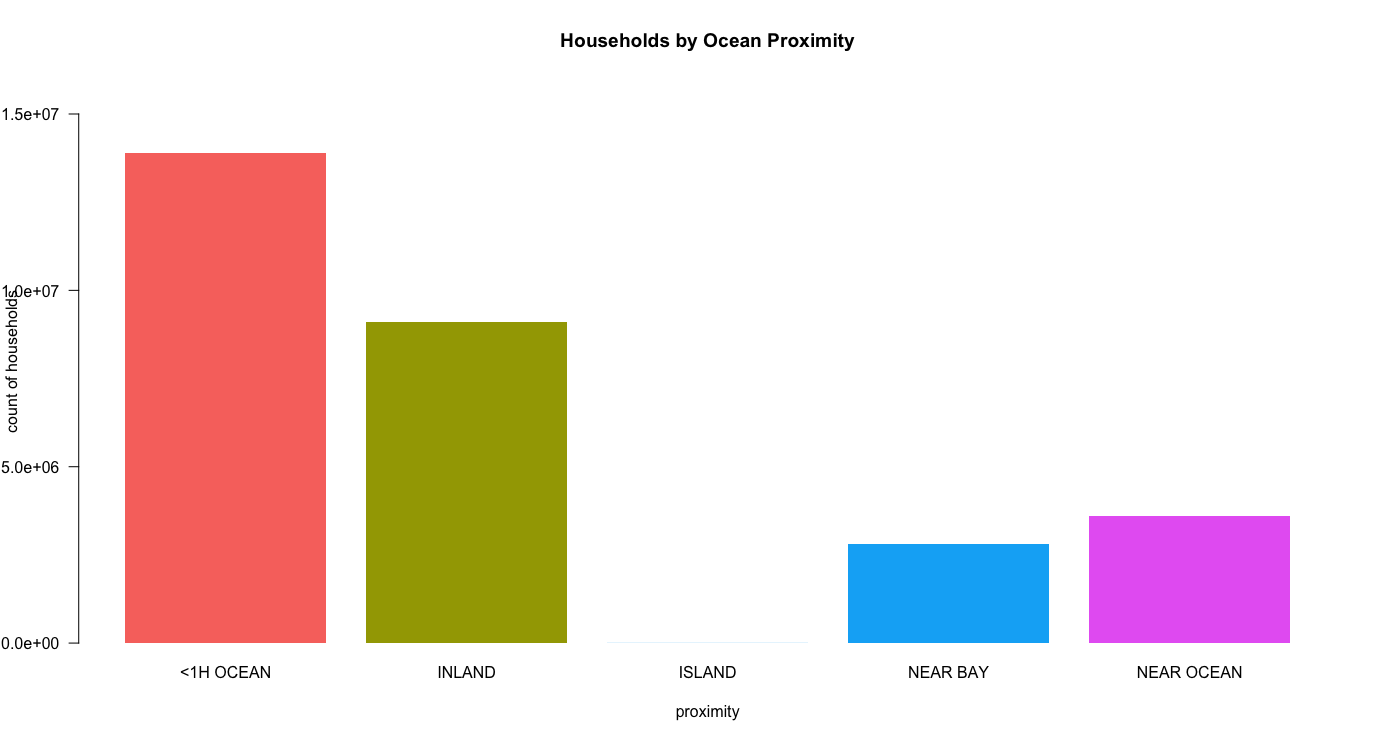This was not a surprise. More households means more people living in that area.

BUT WHY IS THAT? WHY ARE MORE PEOPLE SITUATED AT AREAS THAT ARE 1 HOUR AWAY FROM THE OCEAN AND NOT NEAR THE OCEAN, BAY OR INLAND. LETS FIND OUT.

3. Households by Median Age

``````age_level <- c(0,5,10,15,20,25,30,35,40,45,50,55)
headers <- c("0 to 5","5 to 10", "10 to 15", "15 to 20", "20 to 25","25 to 30",
"30 to 35","35 to 40","40 to 45","45 to 50", "50 to 55")
all_housing <- transform(all_housing, age_cut = cut(housing_median_age, age_level, labels = headers))

barplot( height = rowsum(all_housing\$households,all_housing\$age_cut)[,1]
, las = 1
, col = "#52338B"
, border = NA  # eliminates borders around the bars
, main = "Households by Median Age"
, ylab = "count of households"
, xlab = "Age"
, ylim = c(0,2000000)
)``````
• I wanted to make age group categories as this will help to compress the data and I am not interested in the actual age of the house. Just an age group would be fine to help me understand how old the houses are in those areas.
• First I had to define the cut values for age that will be used as limits for the age groups. "cut(column,cut groups,labels)" function is then used to make the groups of age. "transform" function creates a new column for age group (age_cut) to "all_housing" dataset.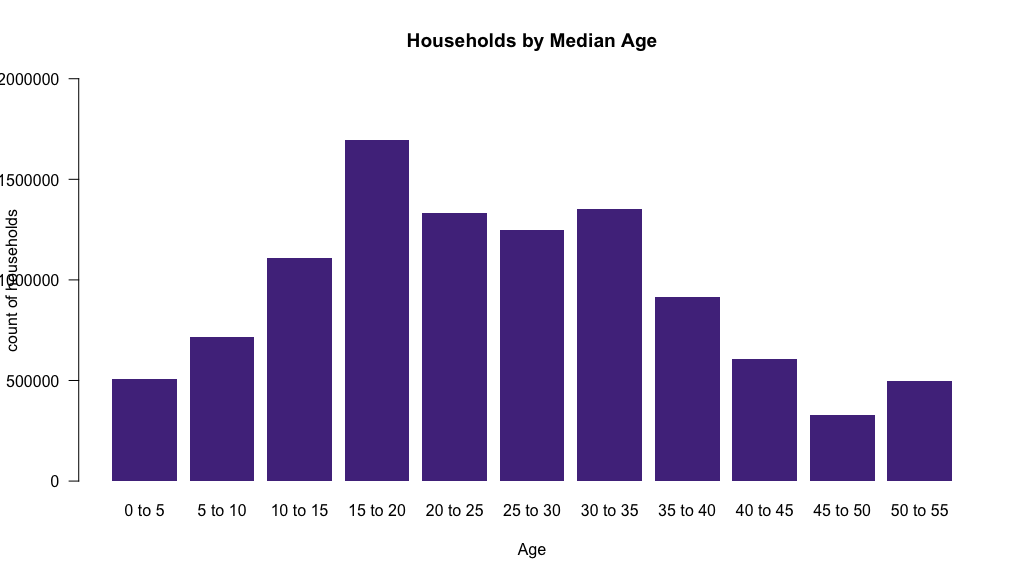The above graph tells us the median age of all the households in California. This looks like a normal distribution where most of the households are 15-35 years old. Let's break it down further by ocean proximity to get a deeper understanding.

``````household_age_1H <- data.frame(matrix(nrow=9136,ncol = 2))
colnames(household_age_1H) <- c("households","agecut")
household_age_1H[,1]<-c(all_housing\$households[all_housing\$ocean_proximity=="<1H OCEAN"])

household_age_inland <- data.frame(matrix(nrow=6551,ncol = 2))
colnames(household_age_inland) <- c("households","agecut")
household_age_inland[,1]<-c(all_housing\$households[all_housing\$ocean_proximity=="INLAND"])

household_age_island <- data.frame(matrix(nrow=5,ncol = 2))
colnames(household_age_island) <- c("households","agecut")
household_age_island[,1]<-c(all_housing\$households[all_housing\$ocean_proximity=="ISLAND"])

household_age_bay <- data.frame(matrix(nrow=2290,ncol = 2))
colnames(household_age_bay) <- c("households","agecut")
household_age_bay[,1]<-c(all_housing\$households[all_housing\$ocean_proximity=="NEAR BAY"])

household_age_ocean <- data.frame(matrix(nrow=2658,ncol = 2))
colnames(household_age_ocean) <- c("households","agecut")
household_age_ocean[,1]<-c(all_housing\$households[all_housing\$ocean_proximity=="NEAR OCEAN"])
• Same as before, I created dataframes for each ocean proximity to store household count for each age group. Each dataframe has 2 columns "households and agecut"
``````barplot( height = rowsum(household_age_1H\$households,household_age_1H\$agecut)[,1]
, las = 1
, col = "#F8756D"
, border = NA  # eliminates borders around the bars
, main = "Households by Median Age (<1H OCEAN)"
, ylab = "count of households"
, xlab = "Age"
, ylim = c(0,800000)
)

barplot( height = rowsum(household_age_inland\$households,household_age_inland\$agecut)[,1]
, las = 1
, col = "#A3A500"
, border = NA  # eliminates borders around the bars
, main = "Households by Median Age (INLAND)"
, ylab = "count of households"
, xlab = "Age"
, ylim = c(0,800000)
)

barplot( height = rowsum(household_age_island\$households,household_age_island\$agecut)[,1]
, las = 1
, col = "#03B0F6"
, border = NA  # eliminates borders around the bars
, main = "Households by Median Age (ISLAND)"
, ylab = "count of households"
, xlab = "Age"
, ylim = c(0,800000)
)

barplot( height = rowsum(household_age_bay\$households,household_age_bay\$agecut)[,1]
, las = 1
, col = "#00BE7D"
, border = NA  # eliminates borders around the bars
, main = "Households by Median Age(NEAR BAY)"
, ylab = "count of households"
, xlab = "Age"
, ylim = c(0,800000)
)

barplot( height = rowsum(household_age_ocean\$households,household_age_ocean\$agecut)[,1]
, las = 1
, col = "#E76AF3"
, border = NA  # eliminates borders around the bars
, main = "Households by Median Age (NEAR OCEAN)"
, ylab = "count of households"
, xlab = "Age"
, ylim = c(0,800000)
)``````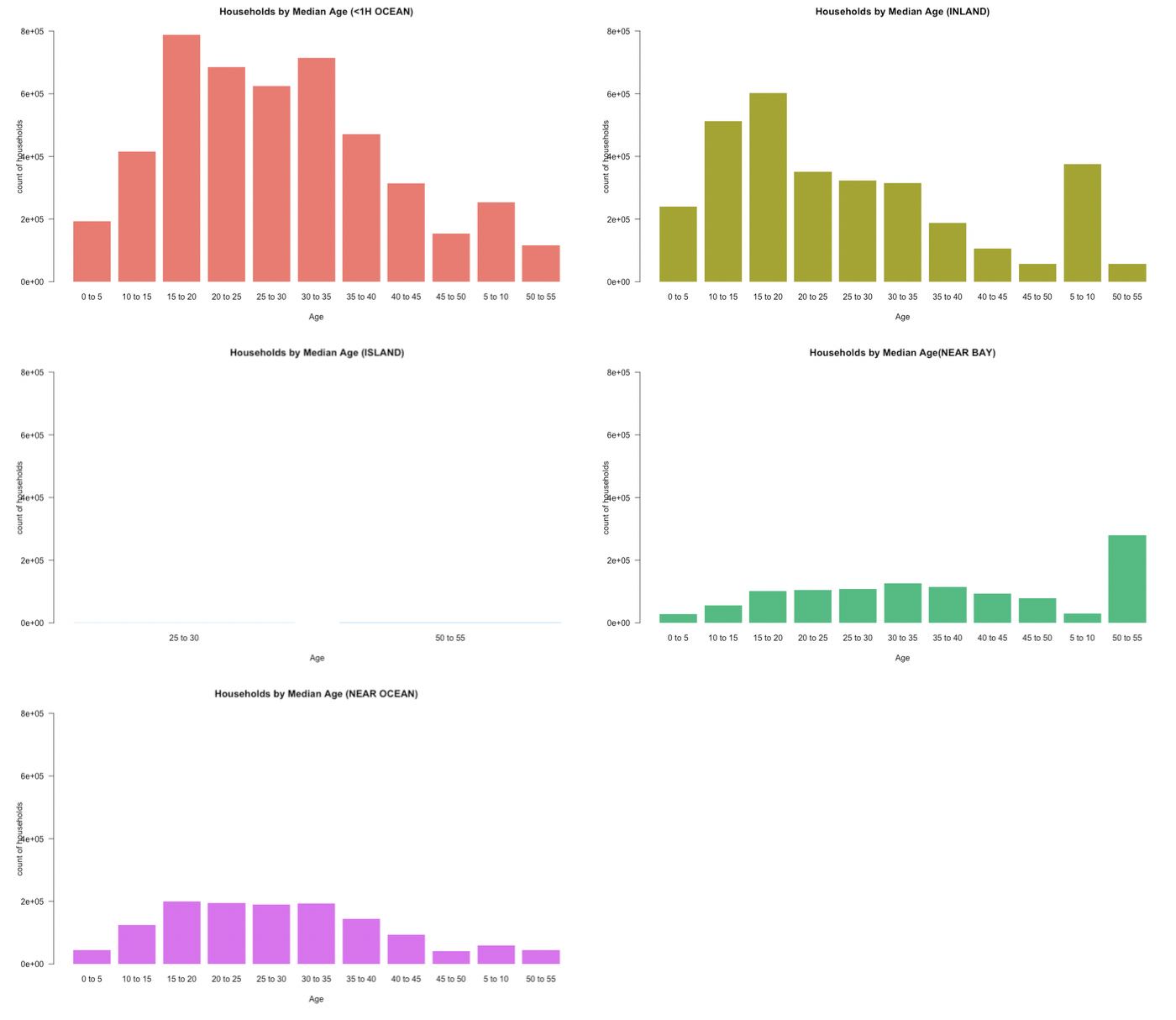All graphs except "Near Bay" have the more or less same distribution of households with most of them being in the age group od 10-40 years old. This means that construction of new houses are quiet common in these areas.

Now, If I combine this data of median age of households with the population data. It can be infered/assumed that most of people living 1hr away from ocean (<1hr OCEAN) moved there around 15-20 years ago.

But what intrigues me is the distribution of "Near bay" graph. unlike the other distributions, this graph shows that most of the households in the bay area are more than 50 years old.

BUT WHY?

On doing further research I found out that its because of San Francisco's old cultural heritage and art.

4. Households by Median Income

``````all_housing\$median_income<-all_housing\$median_income*10000

income_level <- c(0,10000,20000,30000,40000,50000,60000,70000,80000,90000,100000,110000,120000,130000,140000,150000,Inf)
headers_income <- c("0-10k", "10k-20k", "20k-30k","30k-40k", "40k-50k", "50k-60k","60k-70k","70k-80k","80k-90k","90k-100k"
,"100k-110k","110k-120k","120k-130k","130k-140k","140k-150k"," >150k")
all_housing <- transform(all_housing, income_cut = cut(median_income, income_level, labels = headers_income))

household_income_bay <- data.frame(matrix(nrow=2290,ncol = 2))
colnames(household_income_bay) <- c("households","incomecut")
household_income_bay[,1]<-c(all_housing\$households[all_housing\$ocean_proximity=="NEAR BAY"])

household_income_ocean <- data.frame(matrix(nrow=2658,ncol = 2))
colnames(household_income_ocean) <- c("households","incomecut")
household_income_ocean[,1]<-c(all_housing\$households[all_housing\$ocean_proximity=="NEAR OCEAN"])

household_income_1H <- data.frame(matrix(nrow=9136,ncol = 2))
colnames(household_income_1H) <- c("households","incomecut")
household_income_1H[,1]<-c(all_housing\$households[all_housing\$ocean_proximity=="<1H OCEAN"])

household_income_inland <- data.frame(matrix(nrow=6551,ncol = 2))
colnames(household_income_inland) <- c("households","incomecut")
household_income_inland[,1]<-c(all_housing\$households[all_housing\$ocean_proximity=="INLAND"])

household_income_island <- data.frame(matrix(nrow=5,ncol = 2))
colnames(household_income_island) <- c("households","incomecut")
household_income_island[,1]<-c(all_housing\$households[all_housing\$ocean_proximity=="ISLAND"])
``````barplot( height = rowsum(all_housing\$households,all_housing\$income_cut)[,1]
, las = 1
, col = "#52338B"
, border = NA  # eliminates borders around the bars
, main = "Households by Median Income"
, ylab = "count of households"
, xlab = "Income"
, ylim = c(0,3000000)
, space = 0.07
)``````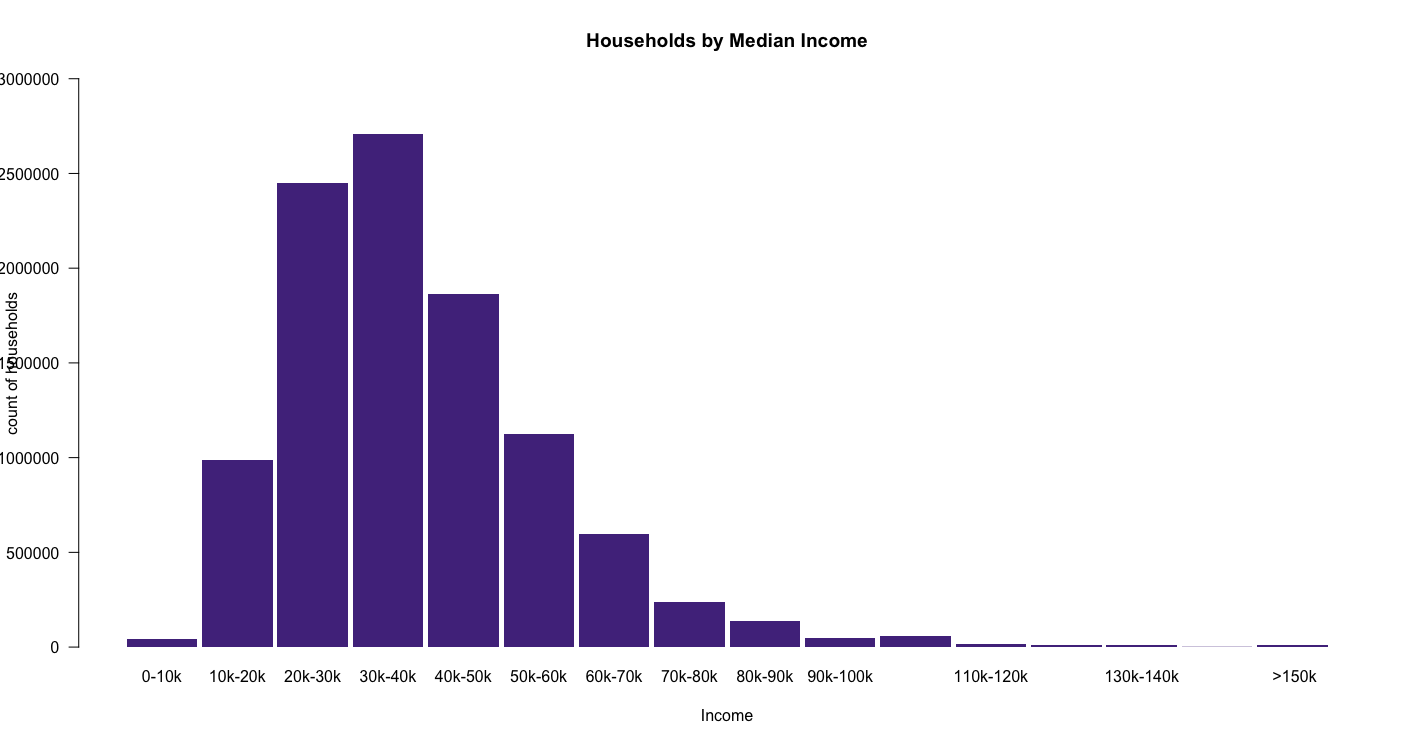This graph talks about the median income of the people living in a household. This will give us an idea of the price/rent of the houses they live in.

On analysis, it is clear that median income of most of the households is between \$20k to \$50k and there are very few households who have their median income above \$100K.

Now, let's look at this based on ocean proximity.

``````barplot( height = rowsum(household_income_1H\$households,household_income_1H\$incomecut)[,1][headers_income]
, las = 1
, col = "#F8756D"
, border = NA  # eliminates borders around the bars
, main = "Households by Median Income (<1H OCEAN)"
, ylab = "count of households"
, xlab = "Age"
, ylim = c(0,1400000)
)

, las = 1
, col = "#A3A500"
, border = NA  # eliminates borders around the bars
, main = "Households by Median Income (INLAND)"
, ylab = "count of households"
, xlab = "Age"
, ylim = c(0,1400000)
)

, las = 1
, col = "#03B0F6"
, border = NA  # eliminates borders around the bars
, main = "Households by Median Income (ISLAND)"
, ylab = "count of households"
, xlab = "Age"
, ylim = c(0,1400000)
)

, las = 1
, col = "#00BE7D"
, border = NA  # eliminates borders around the bars
, main = "Households by Median Income (NEAR BAY)"
, ylab = "count of households"
, xlab = "Age"
, ylim = c(0,1400000)
)

, las = 1
, col = "#E76AF3"
, border = NA  # eliminates borders around the bars
, main = "Households by Median Income (NEAR OCEAN)"
, ylab = "count of households"
, xlab = "Age"
, ylim = c(0,1400000)
)``````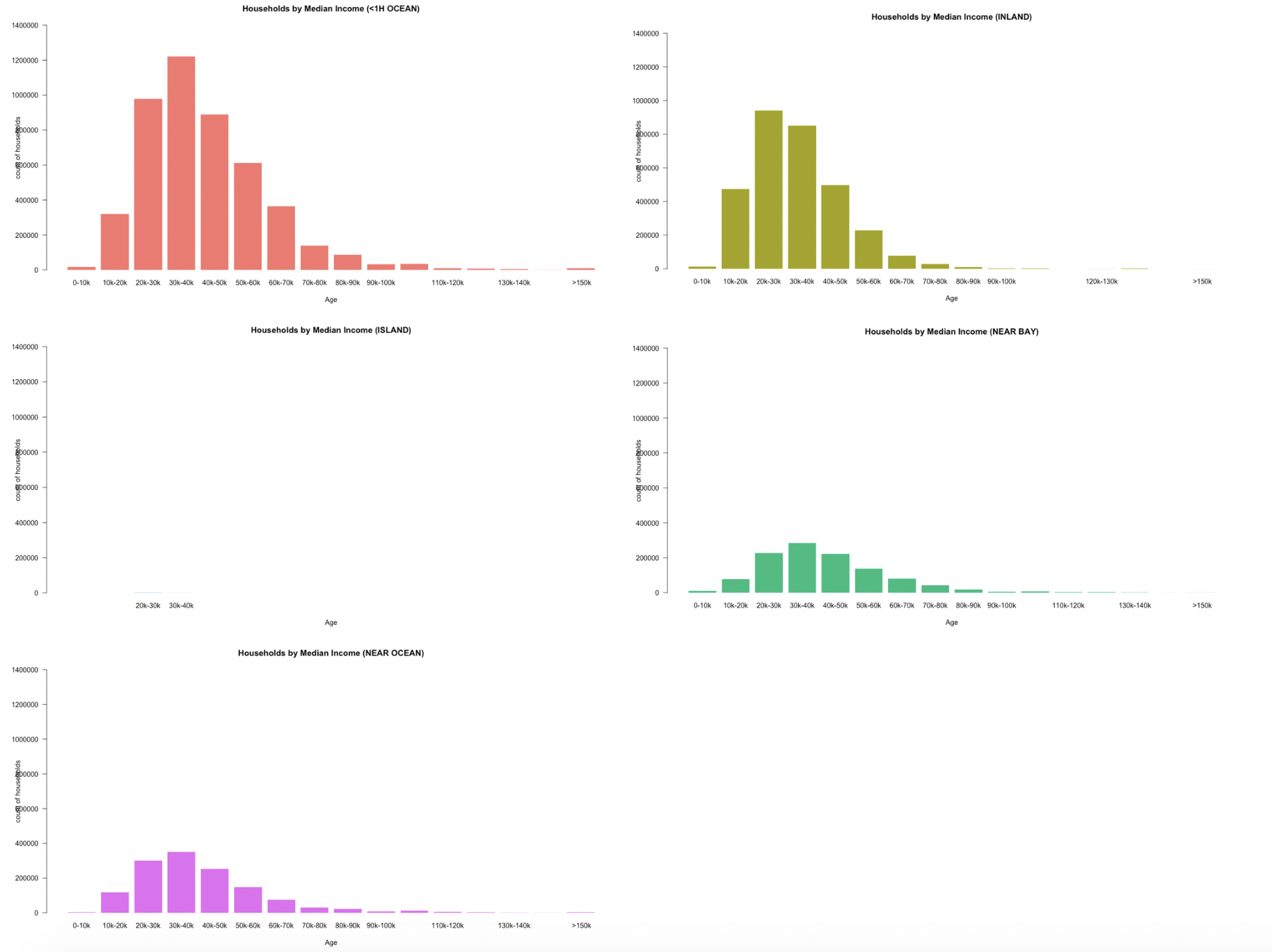Most of them follow the same trend, haveing median income in \$30K - \$40K range except households in inland (INLAND). Median income of households in inland are in the \$20k - \$30k, which is quiet obvious as it is away from the ocean and expense of living is less and hence income is less.

# Pricing Analysis

I wanted to understand the different factors that effect the price of a house

I found making of the bar plots to be repetative so decided to go for a different visualization method which is easier to understand at the same time less work for me to make these graphs

1. Median Price by Ocean Proximity
``````stack_plot <- ggplot(data = all_housing) + geom_bar(map = aes(x = all_housing\$value_cut,
fill = all_housing\$ocean_proximity))

stack_plot + labs(x="price range",y="count of households",title="Median price of households") +
labs(colour = "ocean proximity")``````

- I used ggplot library to construct stack plot. stack plot is particularly useful if you want to show relationship between 3 parameters. In this case, x-axis is the range of values (range-cut), y-axis is the count or frequency (count of households), and the colors filled inside the bars are the ocean proximity. - second line of code lets you add labels to your stackplot.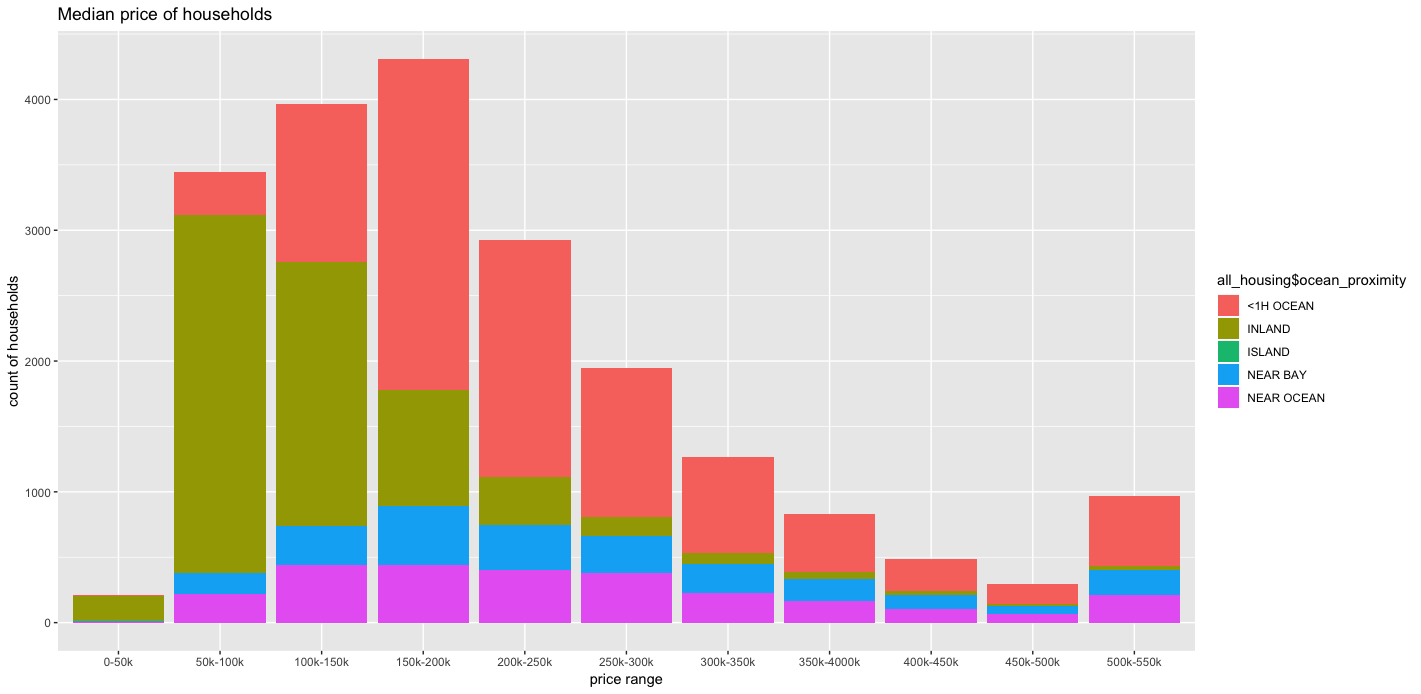It can be seen from the stackplot that most of the houses that are cheap are inland. As we come closer to the ocean the price of the houses increases (green color blocks gets narrower as the price range increases). There are a lot of houses of all price range that are 1 hour away from the ocean (<1h ocean). But there are little to no houses that are in the range of \$0-\$50k except inland.

Now I want to know the exact location of these households based on their price.

``````rCols <- heat.colors(12,0.25)
brCols <- rCols[all_housing\$value_cut]

plot(us.states, xlim=xlim, ylim=ylim,main="median price by ocean proximity")
points(all_housing\$longitude,all_housing\$latitude,col=brCols,pch = 18, cex = 0.4)``````

- heat.colors(n,alpha) function is used to define the colors for the heat map . "12" is the number of colors I needed and "0.25" is the value of alpha or transparency. "brCols" assigns "rcol" to the "value_cut"

- Then I constructed the map using plot function as I did prviously and used points function to plot the points on the map. I used "brcol" in the "col" attribute of the function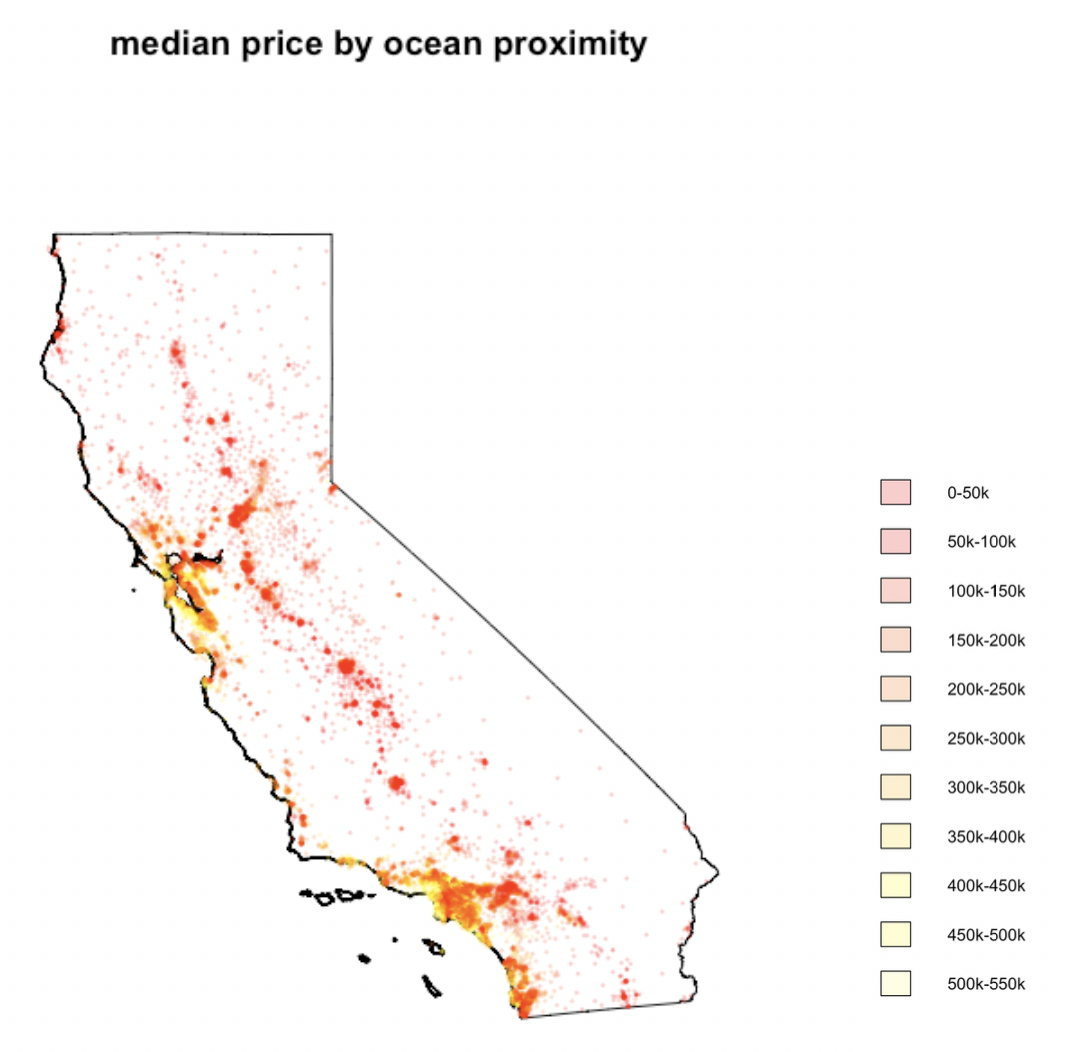This map depicts the location of houses based on their prices. The points that are red in colour are inexpensive and are mostly located inland as we know from the stackplot. The points that are yellow anr expensive. Brighter colors tell us that those areas are densely populated with houses with same price range. So it can be seen that inland have only red spots. Bay area and areas near the ocean have both yellow and red and colors ranging between yellow and red. this means that these areas have houses in almost all price range.

``````plot(us.states, xlim=xlim, ylim=ylim,main="median price by ocean proximity (NEAR BAY)")
points(all_housing\$longitude[all_housing\$ocean_proximity=="NEAR BAY"],all_housing\$latitude[all_housing\$ocean_proximity=="NEAR BAY"],col=brCols,pch = 18, cex = 0.4)

plot(us.states, xlim=xlim, ylim=ylim,main="median price by ocean proximity (NEAR OCEAN)")
points(all_housing\$longitude[all_housing\$ocean_proximity=="NEAR OCEAN"],all_housing\$latitude[all_housing\$ocean_proximity=="NEAR OCEAN"],col=brCols,pch = 18, cex = 0.2)

plot(us.states, xlim=xlim, ylim=ylim,main="median price by ocean proximity (INLAND)")
points(all_housing\$longitude[all_housing\$ocean_proximity=="INLAND"],all_housing\$latitude[all_housing\$ocean_proximity=="INLAND"],col=brCols,pch = 18, cex = 0.2)

plot(us.states, xlim=xlim, ylim=ylim,main="median price by ocean proximity (ISLAND)")
points(all_housing\$longitude[all_housing\$ocean_proximity=="ISLAND"],all_housing\$latitude[all_housing\$ocean_proximity=="ISLAND"],col=brCols,pch = 18, cex = 1.8)

plot(us.states, xlim=xlim, ylim=ylim,main="median price by ocean proximity (<1H OCEAN)")
points(all_housing\$longitude[all_housing\$ocean_proximity=="<1H OCEAN"],all_housing\$latitude[all_housing\$ocean_proximity=="<1H OCEAN"],col=brCols,pch = 18, cex = 0.2)

legend(legend = 1:12, fill = rCols, "topright")``````

Constructed the same map for each ocean proximity seperately.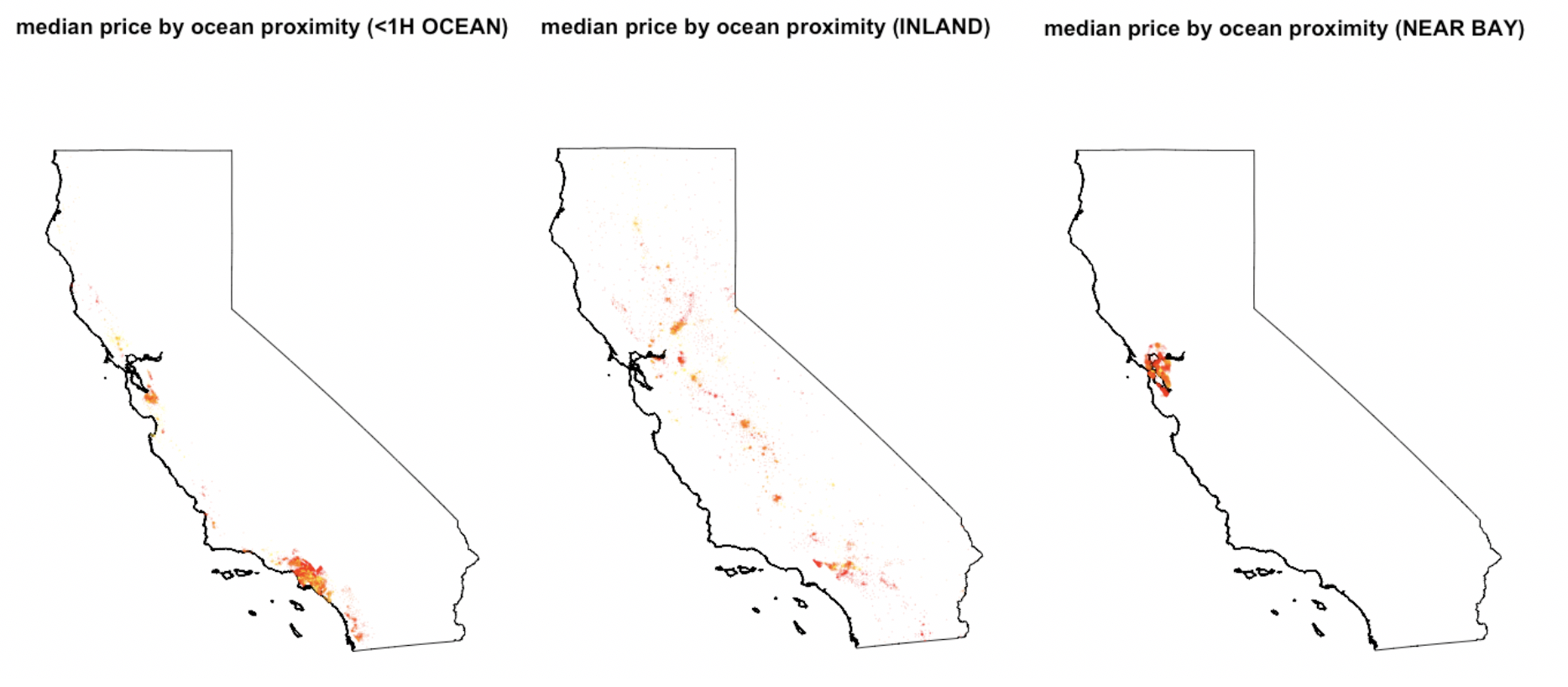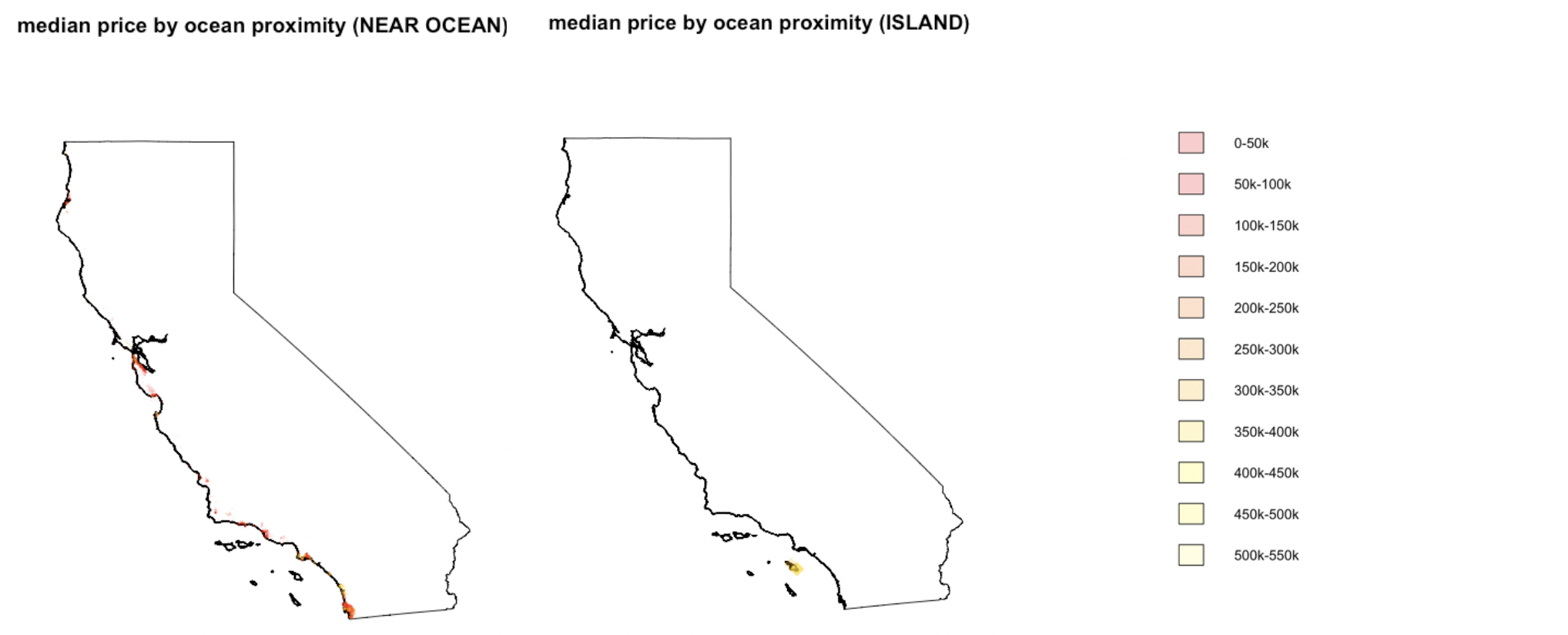Though I got a lot of information from this dataset and learned about the San Francisco housing situation, it was not enough to help me understand different factors that effect the pricing in this state. Factors like occupation, travel time to work, school, stores,etc. I will be on a look out to find a better dataset that would help me make this analysis more richer.